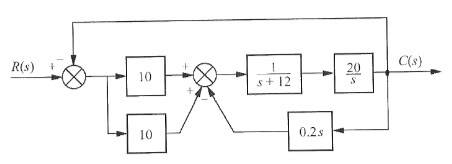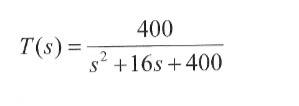# Block diagram reduction

1. Homework Statement2. Homework Equations

I need to reduce the above block diagram to find the closed loop transfer function

This is my solution to the reduction part of the problem:

i) Add the feed forward paths; (10 + 10)

ii) cascade G(s)2 x G(s)3 , {G(s)2 = 1/1+12, G(s)3= 20/s}

iii) Reduce the minor feedback loop (0.2s) : using, G(s)/1+G(s)H(s) [G(s) being part ii]

iv) then finally cascade blocks in forward path

3. The Attempt at a Solution

This should be my open loop transfer function and from there I can get closed loop BUT i'm getting something wrong in my above reduction which I can't figure out. Anyone see where i'm going wrong?

## Answers and Replies

Can anyone help me out

this is the answer for the closed loop functionfirst convert the 3-input summation into two 2-input summation.
and after that i will become very easy to solve

This question was asked in May 2011.

Hopefully it was solved by now.

But yes I agree with trapti once you separate the middle summing junction into two it is very simple.